Practice
Resources
Contests
Online IDE
New
Free Mock
Scaler
Practice
Improve your coding skills with our resources
Contests
Compete in popular contests with top coders
Scaler
Explore Offerings by SCALER## Welcome to Interviewbit, help us create the best experience for you!

Currently, You are a:College/University *
Enter the name of your college
Branch *
Year of completion *College/University *
Enter the name of your college
Branch *
Year of completion *Current Company *
Enter company name
Experience *## You're all set!Full name *
Email *

By creating an account, I acknowledge that I have read and agree to InterviewBit’s Terms and Privacy Policy .## Welcome back!

Email *Go to Problems

Be a Code Ninja!

# Bubble Sort

Bubble sort, also referred to as comparison sort, is a simple sorting algorithm that repeatedly goes through the list, compares adjacent elements and swaps them if they are in the wrong order. This is the most simplest algorithm and inefficient at the same time. Yet, it is very much necessary to learn about it as it represents the basic foundations of sorting.

Table of Content

### Application

#### Understand the working of Bubble sort

• Bubble sort is mainly used in educational purposes for helping students understand the foundations of sorting.
• This is used to identify whether the list is already sorted. When the list is already sorted (which is the best-case scenario), the complexity of bubble sort is only `O(n)`.
• In real life, bubble sort can be visualised when people in a queue wanting to be standing in a height wise sorted manner swap their positions among themselves until everyone is standing based on increasing order of heights.

### Explanation

Algorithm: We compare adjacent elements and see if their order is wrong (i.e `a[i] > a[j] for 1 <= i < j <= size of array`; if array is to be in ascending order, and vice-versa). If yes, then swap them.

Explanation:

• Let us say, we have an array of length `n`. To sort this array we do the above step (swapping) for `n - 1` passes.
• In simple terms, first, the largest element goes at its extreme right place then, second largest to the last by one place, and so on. In the ith pass, the ith largest element goes at its right place in the array by swappings.
• In mathematical terms, in `ith` pass, at least one element from `(n - i + 1)` elements from start will go at its right place. That element will be the ith `(for 1 <= i <= n - 1)` largest element of the array. Because in the `ith` pass of the array, in the `jth` iteration `(for 1 <= j <= n - i)`, we are checking `if a[j] > a[j + 1]`, and `a[j]` will always be greater than `a[j + 1]` when it is the largest element in range `[1, n - i + 1]`. Now we will swap it. This will continue until `ith` largest element is at the `(n - i + 1)`th position of the array.

### Bubble Sort Example:

Consider the following array: Arr=`14, 33, 27, 35, 10`. We need to sort this array using bubble sort algorithm.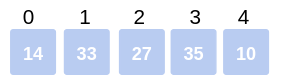First Pass:

• We proceed with the first and second element i.e., Arr and Arr. Check if `14 > 33` which is false. So, no swapping happens and the array remains the same.• We proceed with the second and third element i.e., Arr and Arr. Check if `33 > 27` which is true. So, we swap Arr and Arr.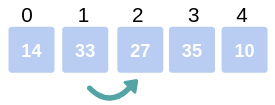Thus the array becomes: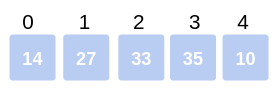• We proceed with the third and fourth element i.e., Arr and Arr. Check if `33 > 35` which is false. So, no swapping happens and the array remains the same.• We proceed with the fourth and fifth element i.e., Arr and Arr. Check if `35 > 10` which is true. So, we swap Arr and Arr.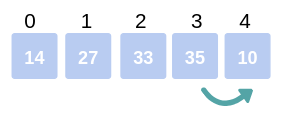Thus, after swapping the array becomes: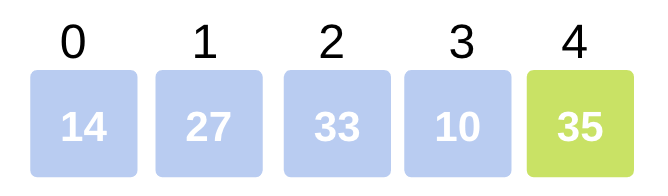Thus, marks the end of the first pass, where the Largest element reaches its final(last) position.

Second Pass:

• We proceed with the first and second element i.e., Arr and Arr. Check if `14 > 27` which is false. So, no swapping happens and the array remains the same.We now proceed with the second and third element i.e., Arr and Arr. Check if 27 > 33 which is false. So, no swapping happens and the array remains the same.

• We now proceed with the third and fourth element i.e., Arr and Arr. Check if `33 > 10` which is true. So, we swap Arr and Arr.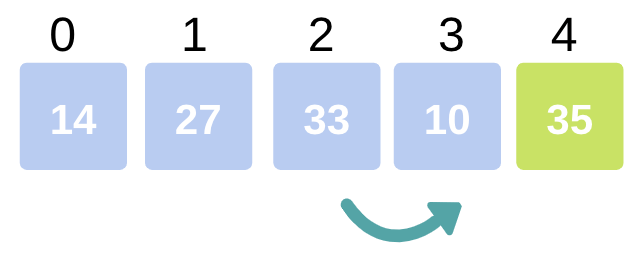Now, the array becomesThus marks the end of second pass where the second largest element in the array has occupied its correct position.

Third Pass:
After the third pass, the third largest element will be at the third last position in the array..

.

i-th Pass:
After the ith pass, the ith largest element will be at the ith last position in the array.

.

.
n-th Pass:
After the nth pass, the nth largest element(smallest element) will be at nth last position(1st position) in the array, where ‘n’ is the size of the array.

After doing all the passes, we can easily see the array will be sorted.
Thus, the sorted array will look like this: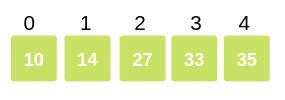### Pseudocode of Bubble Sort Algorithm

```bubbleSort( Arr[], totat_elements)

for i = 0 to total_elements - 1 do:
swapped = false

for j = 0 to total_elements - i - 2 do:

/* compare the adjacent elements */
if Arr[j] > Arr[j+1] then
/* swap them */
swap(Arr[j], Arr[j+1])
swapped = true
end if

end for

/*if no number was swapped that means
array is sorted now, break the loop.*/

if(not swapped) then
break
end if

end for

end
```

## Implementation Of Bubble Sort

``````Implementation of bubble sort in C
#include<stdio.h>

int main()
{
int arr[] = {5, 6, 2 ,6, 9, 0, -1}, n = 7, i, j;

for(i = 0; i < n - 1; ++i)
{
int swapped = 0;

for(j = 0; j < (n - i - 1); ++j)
if(arr[j] > arr[j+1])
{
int temp = arr[j];
arr[j] = arr[j+1];
arr[j+1] = temp;

swapped = 1;
}
if(!swapped)
break;
}

printf("\nArray after sorting: ");
for(i = 0; i < n; ++i)
printf("%d ", arr[i]);

return 0;
}``````
``````Implementation of Bubble sort in C++
#include <iostream>
#include <vector>

using namespace std;

void BubbleSort (vector<int> &arr, int n)
{
for (int i = 0; i < n - 1; ++i)
{
bool swapped = false;
for (int j = 0; j < n - i - 1; ++j)
{
if (arr[j] > arr[j+1]) //check if adjacent element is
//not in order
{
swap(arr[j], arr[j + 1]);
swapped = true;
}
}
// Value at n-i-1 will be maximum of all the values below this index.

if(!swapped)
break;
}
}

int main()
{
vector<int> arr = {5, 6, 2, 6, 9, 0, -1};
int n = 7;

BubbleSort(arr, n);

// Display the sorted data.
cout << "\nSorted Data: ";
for (i = 0; i < n; i++)
Cout << arr[i] << “ “;

return 0;
}``````
``````Implementation of Bubble Sort in Java:
import java.util.Scanner;
class BubbleSort {
public static void main(String []args) {
int n;
Scanner in = new Scanner(System.in);
System.out.println("Input number of integers to sort");
n = in.nextInt();
int array[] = new int[n];
System.out.println("Enter " + n + " integers");
for (int i = 0; i < n; i++)
array[i] = in.nextInt();

for (int i = 0; i < n - 1; i++) {
Boolean swapped = false;
for (int j = 0; j < n - i - 1; j++) {
if (array[j] > array[j+1]) /* For descending order use < */
{
int temp = array[j];
array[j]= array[j+1];
array[j+1] = temp;

swapped = true;
}
}
if(!swapped)
break;
}
System.out.println("Sorted list of numbers:");
for (int i = 0; i < n; i++)
System.out.println(array[i]);
}
}``````
``````Implementation of bubble sort in python
def bubble_sort(arr):
for i in range(len(arr) - 1):
swapped = False
for j in range(0, len(arr) - i - 1):
if arr[j] > arr[j + 1]:
arr[j], arr[j + 1] = arr[j + 1], arr[j]
swapped = True
if not swapped:
return

arr = [int(x) for x in input(‘Enter numbers: ’).split()]
bubble_sort(arr)
print('Sorted list: ', end='')
print(arr)``````

### Complexity Analysis

#### Time Complexity of Bubble sort

• Best case scenario: The best case scenario occurs when the array is already sorted. In this case, no swapping will happen in the first iteration (The `swapped` variable will be false). So, when this happens, we break from the loop after the very first iteration. Hence, time complexity in the best case scenario is `O(n)` because it has to traverse through all the elements once.
• Worst case and Average case scenario: In Bubble Sort, `n-1` comparisons are done in the 1st pass, `n-2` in 2nd pass, `n-3` in 3rd pass and so on. So, the total number of comparisons will be:
``````Sum = (n-1) + (n-2) + (n-3) + ..... + 3 + 2 + 1
Sum = n(n-1)/2
``````
Hence, the time complexity is of the order n2 or O(n2).

#### Space Complexity of Bubble sort

The space complexity for the algorithm is O(1), because only a single additional memory space is required i.e. for temporary variable used for swapping.

### FAQs

• What is the best case time complexity of bubble sort?
The time complexity in the best case scenario is `O(n)` because it has to traverse through all the elements once to recognize that the array is already sorted.

• What is the advantage of bubble sort over other sorting techniques?

• The built-in ability to detect whether the list is sorted efficiently is the only advantage of bubble sort over other sorting techniques.
• When the list is already sorted (which is the best-case scenario), the complexity of bubble sort is only `O(n)`.
• It is faster than other in case of sorted array and consumes less time to describe whether the input array is sorted or not.
• Why bubble sort is called “bubble” sort?
The “bubble” sort is called so because the list elements with greater value than their surrounding elements “bubble” towards the end of the list. For example, after first pass, the largest element is bubbled towards the right most position. After second pass, the second largest element is bubbled towards the second last position in the list and so on.

• Is bubble sort a stable algorithm?

• Bubble sort is a stable algorithm.
• A sorting algorithm is said to be stable if two objects with equal keys appear in the same order in sorted output as they appear in the input array to be sorted.
• Is bubble sort an inplace algorithm?

• Bubble sort is an inplace algorithm.
• An algorithm is said to be inplace if it does not need an extra space and produces an output in the same memory that contains the data by transforming the input ‘in-place’. However, a small constant extra space used for variables is allowed.
• Is Bubble sort slow?

• Bubble sort is slower than the other O(n2) sorting algorithms.
• It is about four times as slow as insertion sort and twice as slow as selection sort.
• It has a good best-case behavior, but is impractically slow on almost all real world data sets and is not considered for implementation in real applications.
• Can bubble sort be implemented recursively?

• Yes.
• Recursive Bubble Sort has no additional performance/implementation advantages, but can be used to understand recursion and sorting concepts better.
• Base Case: If array size is 1, return.
• Do One Pass of normal Bubble Sort. This pass bubbles largest element of current subarray to correct position.
• Recur for all elements except last of current subarray.
``````    recursiveBubbleSort(arr[], n){
// Base case
if (n == 1)
return;

// One pass of bubble sort. After
// this pass, the largest element
// is moved (or bubbled) to end.
for(i=0; i<n-1; i++){
if(arr[i] > arr[i+1])
{
swap(arr[i], arr[i+1]);
}
}

// recursion for remaining elements in array
recursiveBubbleSort(arr, n-1);
}``````

## Serious about Learning Programming ?

Learn this and a lot more with Scaler Academy's industry vetted curriculum which covers Data Structures & Algorithms in depth.
Primers
Examples

## Arrays Problems

Array math
Value ranges
Problem Score Companies Time Status
Max Min 150
17:31
Merge Intervals 225 78:57
Merge Overlapping Intervals 225 48:24
Simulation array
Problem Score Companies Time Status
Perfect Peak of Array 200 48:49
Kth Row of Pascal's Triangle 225
28:32
Spiral Order Matrix II 225 48:40
Pascal Triangle 225 26:46
Anti Diagonals 225 41:46
Bucketing
Problem Score Companies Time Status
Triplets with Sum between given range 200
76:05
Balance Array 200 61:31
Find Duplicate in Array 450 40:13
Maximum Consecutive Gap 450 58:46
Arrangement
Problem Score Companies Time Status
Sort array with squares! 200 31:16
Largest Number 225 70:26
Rotate Matrix 300 60:26
Next Permutation 300 63:13
Find Permutation 300 56:00
Sorting
Problem Score Companies Time Status
Noble Integer 200 43:30
Wave Array 225 22:08
Hotel Bookings Possible 225 66:06
Max Distance 250 68:14
Maximum Unsorted Subarray 250 68:52
Space recycle
Problem Score Companies Time Status
Set Matrix Zeros 300 48:04
First Missing Integer 300 64:38
Maximum Sum Square SubMatrix 300 56:24
Missing / repeated number
Problem Score Companies Time Status
First Missing Integer 300 64:38
Repeat and Missing Number Array 350 63:55
N/3 Repeat Number 600
68:22

Problem Score Companies Time Status
Reorder Data in Log Files 200 42:20
Move Zeroes 200 24:24
Make equal elements Array 200 28:17
Segregate 0s and 1s in an array 200 14:14
Array Sum 200 30:37
Set Intersection 200 60:51
Occurence of Each Number 200 22:32
Greater of Lesser 200 12:03
Spiral Matrix 200 30:23
Product of All 200 29:54
Chips Factory 200 17:43
Greater than All 150 17:04
Pythagorean Triplets 200 22:32
Diagonal Flip 200 16:43
Positive Negative 100 9:33Free Mock Assessment
Help us know you better for the best experience
Current Employer *
Enter company name